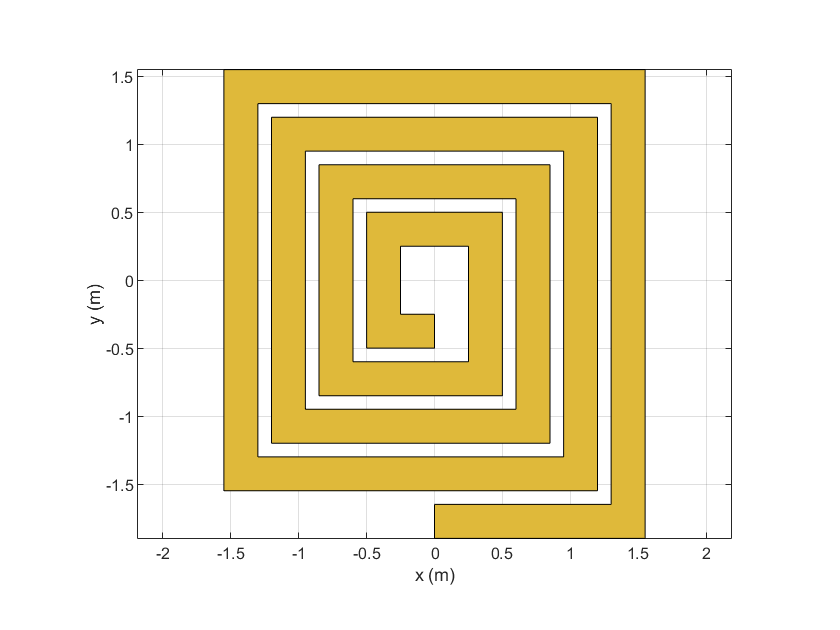# area

Calculate area of RF PCB shape in square meters

Since R2021b

## Syntax

``a = area(shape)``

## Description

example

````a = area(shape)` calculates the area of the shape in units of square meters.```

## Examples

collapse all

Create and view a default spiral trace.

```trace = traceSpiral; show(trace)```Get the area of the spiral trace.

`a = area(trace)`
```a = 4.5200e-04 ```

## Input Arguments

collapse all

RF PCB shape created using custom elements and shape objects of RF PCB Toolbox™, specified as an object.

Example: `shape = bendCurved;` specifies the shape as a `bendCurved` object.

## Version History

Introduced in R2021b